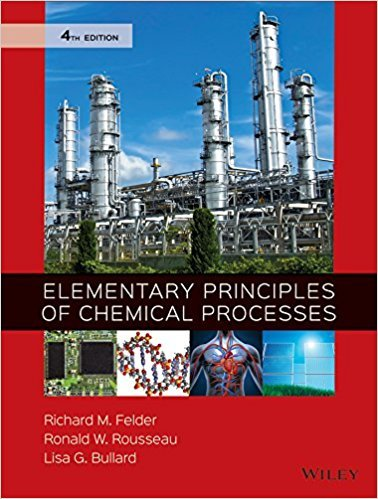×
Get Full Access to Elementary Principles Of Chemical Processes - 4 Edition - Chapter 4.46 - Problem 4.37
Get Full Access to Elementary Principles Of Chemical Processes - 4 Edition - Chapter 4.46 - Problem 4.37

×

# The SO2 scrubbing tower described in 4.36 is to be used toISBN: 9780470616291 248

## Solution for problem 4.37 Chapter 4.46

Elementary Principles of Chemical Processes | 4th Edition

• Textbook Solutions
• 2901 Step-by-step solutions solved by professors and subject experts
• Get 24/7 help from StudySoup virtual teaching assistantsElementary Principles of Chemical Processes | 4th Edition

4 5 1 413 Reviews
12
4
Problem 4.37

The SO2 scrubbing tower described in 4.36 is to be used to reduce the SO2 mole fraction in the waste gas to a level that meets air-quality standards. The solvent feed rate must be large enough to keep the SO2 mass fraction in the exit liquid below a specified maximum value. (a) Draw and label a flowchart of the tower. Along with the molar flow rates and SO2 mole fractions of the four process streams, label the feed gas temperature and pressure [T1(F), P1(psig)], the feed gas orifice meter reading [h1(mm)], the SO2 analyzer reading for the feed gas R1, the volumetric feed rate of solvent to the column V_ 2m3/min, and the SO2 analyzer reading for the exit gasR3. (The temperature and pressure units are taken from the calibration curves for the gauges used to measure these variables.) (b) Derive a series of equations relating all of the labeled variables on the flowchart. The equations should include the calibration formulas calculated in Part (b) of 4.36. Determine the number of degrees of freedom for the process system. In the remainder of the problem, you will be given values of measured feed gas stream variables [T1(F), P1(psig), h1(mm), and R1], the maximum allowed SO2 mole fraction in the exit solution [x4], and the specified SO2 mole fraction in the exit gas (y3), and asked to calculate the expected SO2 analyzer reading for the exit gas R3 and the minimum volumetric flow rate of solvent V_ 2. Part (c) involves using a spreadsheet for the calculation, and Part (d) calls for an independent calculation using an equation-solving program. (c) Create a spreadsheet to store input values of T1, P1, h1, R1, x4, and y3 and to calculate R3 and V_ 2. In the first five rows, insert the values T1 75; P1 150; h1 210; R1 82:4; x4 010, and y3 0:05; 0:025; 0:01; 0:005; and 0:001. In the next five rows, insert the same input values except make x4 0:02. On a single graph, draw plots of V_ 2 versus y3 for each of the two given values of x4 (preferably using the spreadsheet program to generate the graph). Briefly explain the shape of the curves and their position relative to each other. (d) Enter the equations of Part (b) into an equation-solving program. Use the program to calculate R3 and V_ 2 corresponding to T1 75; P1 150; h1 210; R1 82:4; x4 0:10, and y3 0:05; 0:025; It is advisable to solve 4.36 before attempting this problem. ENVIRONMENTAL 186 CHAPTER 4 Fundamentals of Material Balances WEBC04 06/04/2015 20:34:58 Page 187 0:01; 0:005; and 0:001, and then T1 75; P1 150; h1 210; R1 82:4; x4 0:02, and y3 0:05; 0:025; 0:01; 0:005; and 0:001. If you did not already do so in Part (c), draw on a single graph plots of V_ 2 versus y3 for each of the two given values of x4 and briefly explain the shape of the curves and their position relative to each other.

Step-by-Step Solution:
Step 1 of 3

I. Chapter 4: Chemical Quantities and Aqueous Reactions a. Section 4.1: Climate Changing (Not required for exam, just informational) b. Section 4.2: Reactions Stoichiometry i. Stoichiometry: The numerical relationship between chemical amounts in a balanced equation ii. Objective: Calculate mass relationships using balanced chemical equations 1. Example: TiCl (s4 + O (g2  TiO + 22l (g) 2ow many grams of TiO can2be produced from 100.0g of TiCl with excess O 4 2 1molTiCl4 a. 100.0g TiCl x4 189.

Step 2 of 3

Step 3 of 3

##### ISBN: 9780470616291

Unlock Textbook Solution